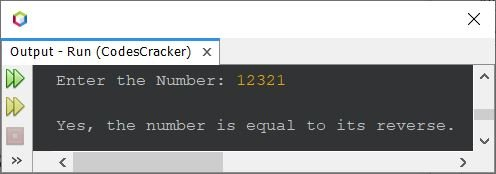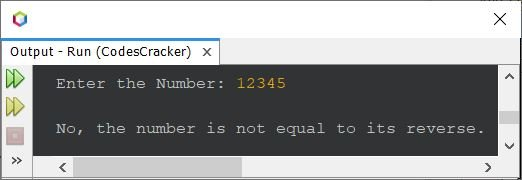# Java Program to Check Original Equal to its Reverse or Not

Other recommended programs, based on this, are:

The question is: write a Java program to check whether a number is equal to its reverse or not. Here is its answer:

```import java.util.Scanner;

public class CodesCracker
{
public static void main(String[] args)
{
int num, orig, rem, rev=0;
Scanner scan = new Scanner(System.in);

System.out.print("Enter the Number: ");
num = scan.nextInt();

orig = num;
while(num>0)
{
rem = num%10;
rev = (rev*10) + rem;
num = num/10;
}

if(orig==rev)
System.out.println("\nYes, the number is equal to its reverse.");
else
System.out.println("\nNo, the number is not equal to its reverse.");
}
}```

The snapshot given below shows the sample run of the above program with user input 12321:Here is another sample run with user input 12345:The program starts by importing the Scanner class from the java.util package, which is used to read user input.

The program defines a public class called CodesCracker with a public static method called main that takes an array of strings as input.

The main method initializes four variables of type int (num, orig, rem, and rev) to store the user input number, the original number, the remainder of the number, and the reversed number, respectively.

The program creates a new Scanner object called scan to read user input from the console.

The program prompts the user to enter a number using the print() method of the System.out object and stores the user's input in the variable num using the nextInt() method of the Scanner object.

The program assigns the value of num to orig, so that the original number can be compared to its reverse later.

The program uses a while loop to reverse the digits of the number. The loop continues until num becomes 0. Inside the loop, the remainder of num is calculated by multiplying the modulus by 10, and this remainder is added to rev multiplied by 10. Finally, num is divided by 10 to remove the digit that has been processed.

After the loop finishes, the program checks if the original number is equal to its reverse by comparing orig with rev. If the two values are equal, the program prints a message indicating that the number is equal to its reverse. Otherwise, the program prints a message indicating that the number is not equal to its reverse.

Java Online Test

« Previous Program Next Program »